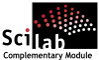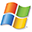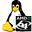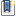ATOMS : Accsum details

# Accsum

Accurate summation algorithmsDetails
Version
0.1.2
Author
Michael Baudin
Owner Organization
DIGITEO
Maintainers
Michael BAUDIN
Antoine ELIAS
Category
Dependencies
Creation Date
July 17, 2014
Source created on
Scilab 5.5.x
Binaries available on
Scilab 5.5.x:Linux 32-bitWindows 32-bitWindows 64-bitmacOSLinux 64-bit
Install command
`--> atomsInstall("accsum")`
Report a bugDescription
```            The goal of this toolbox is to provide accurate algorithms to
compute sums.
We consider here sums of a given dataset x, that is, we
consider s = x(1)+x(2)+...+x(n).

These algorithms may be required to manage datasets which
are ill-conditionned with respect to the sum function, which
happens when the data are varying highly in magnitude and in
sign.
Hence, these datasets are very sensitive to small changes in the
input.
In this case, the "sum" function of Scilab is not appropriate
and may produce results which have only a small number of significant
digits, or no significant digit at all.
Users may consider the condnum module and the condnb_sumcond
function to compute the condition number of a particular sum.
See http://atoms.scilab.org/toolboxes/condnb
for details.

The flagship of this module is the accsum_fdcs function, which
provides a doubly self compensated sum algorithm.
This function is based on compiled source code, so that it is
fast enough, even for relatively large datasets.
The data must be ordered in decreasing magnitude.
To do this, we may use the accsum_order function with order=5.

The module is mainly based on the book "Stability and numerical
accuracy of algorithms" by Nicolas Higham.

The toolbox is based on macros and compiled source code.

Type "help accsum_overview" for quick start.

Features
--------

The following is a list of the current accsum functions :
* accsum_dcs : A Doubly Self Compensated Sum algorithm
* accsum_scs : A Self Compensated Sum algorithm
* accsum_compsum : The compensated sum of a matrix.
* accsum_dblcompsum : The doubly compensated sum of a matrix.
* accsum_fasttwosum : The fast2sum sum of a and b.
* accsum_orderdynamic : Returns the sum with a dynamic re-ordering.
* accsum_straight : The straightforward sum of a matrix.
* accsum_twosum : The twosum sum of a and b.
* accsum_fcompsum : The compensated sum of a matrix.
* accsum_fdcs : A Doubly Self Compensated Sum algorithm
* accsum_fscs : A Self Compensated Sum algorithm

and support functions:

* accsum_getpath : Returns the path to the current module.
* accsum_order : Re-order the matrix.
* accsum_priestx : A difficult example for SCS by Priest.
* accsum_shuffle : Randomly shuffles the input.
* accsum_sumcond : Condition number of the sum function.
* accsum_wilkinson : A test vector by Wilkinson.
* accsum_higham : Returns an example designed by Higham.

The accsum_fcompsum, accsum_fdcs and accsum_fscs functions
are based on compiled source code and are faster than the other.

Bibliography
------------
* "Stability and numerical accuracy of algorithms", Nicolas Higham
* "Handbook of Floating Point Computations", Muller et al
* https://hpcrd.lbl.gov/SCG/ocean/NRS/ECMWF/img14.htm
* https://hpcrd.lbl.gov/SCG/ocean/NRS/SCSsum.F
* "On properties of floating point arithmetics: numerical stability and the
cost of accurate computations", Douglas Priest, 1992
* "Using Accurate Arithmetics to Improve Numerical Reproducibility and
Stability in Parallel Applications". Yun He and Chris H.Q. Ding.  Journal of
Supercomputing,  Vol.18, Issue 3, 259-277, March 2001. Also Proceedings of
International Conference  on Supercomputing (ICS'00), May 2000, 225-234.

```Files (6)Source code archive
```Same sources as in previous toolbox version, updated to make it available for
Scilab 5.5.0
```Linux 32-bit binary for Scilab 5.5.x
```Linux 32-bit
Automatically generated by the ATOMS compilation chain

```Windows 32-bit binary for Scilab 5.5.x
```Windows 32-bit
Automatically generated by the ATOMS compilation chain

```Windows 64-bit binary for Scilab 5.5.x
```Windows 64-bit
Automatically generated by the ATOMS compilation chain

```macOS binary for Scilab 5.5.x
```MacOSX version
Automatically generated by the ATOMS compilation chain

```Linux 64-bit binary for Scilab 5.5.x
```Linux 64-bit
Automatically generated by the ATOMS compilation chain

```News (0)Comments (0)Leave a comment
Login withEmail notifications